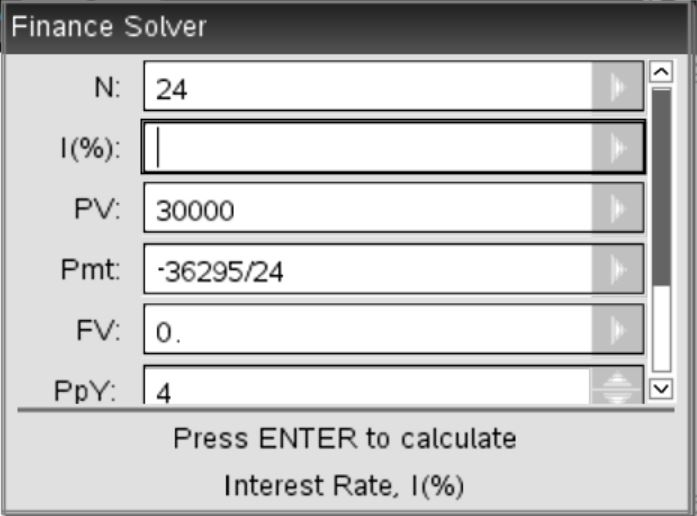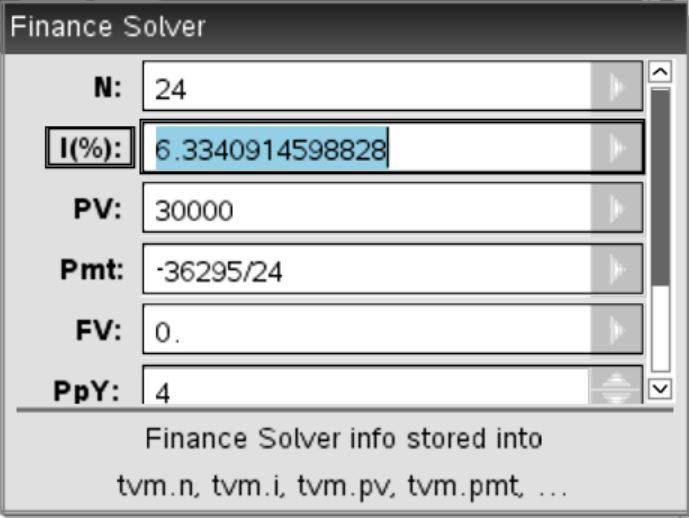# Finance loan interest rate homework

Homework Statement:
A loan of $30,000 is paid back after 6 years with a final value of$36,295. At what interest rate, compounding quarterly, has this money been invested?
Relevant Equations:
Finance solver
I got 6.33% but apparently it's 3.2%

Gold Member
2022 Award
And are we supposed to guess how you got that? I suppose we could just speculate about where you went wrong (or didn't) but it seems like a waste of time. How about you show your work?Homework Helper
2022 Award
Problem Statement: A loan of $30,000 is paid back after 6 years with a final value of$36,295. At what interest rate, compounding quarterly, has this money been invested?
Relevant Equations: Finance solver

I got 6.33% but apparently it's 3.2%

If an amount $A$ is invested for $N$ years at a rate $r\,\%$ and compounded quarterly, then the final value is $$F = A\left(1 + \frac{r}{400}\right)^{4N}.$$ You are given $F$, $N$ and $A$ and asked to solve for $r$.

•HallsofIvy and PeroK
Mentor
@paddo, what does the solver do if you enter 6 for N instead of 24? I'm thinking that N represents the number of years, not the number of payments. There is already a field for the number of payments per year (PpY). The interest rate that I get by direct calculation is a little under 3.2%.

Homework Helper
2022 Award
@paddo, what does the solver do if you enter 6 for N instead of 24? I'm thinking that N represents the number of years, not the number of payments. There is already a field for the number of payments per year (PpY). The interest rate that I get by direct calculation is a little under 3.2%.

I don't think that solver is designed to deal with the situation in the OP in any event.

I think it's designed to deal with the situation where regular repayments are made throughout the term, thereby reducing the balance on which interest is charged. The OP suggests instead a single payment at the end of the term.

If an amount $P$ is lent at a rate of $r\,\%$ to repaid by $n$ equal installments of $A$ per year over $Y$ years, then the balance outstanding after $k+1$ periods is $$B_{k+1} = B_k\left(1 + \frac{r}{100n}\right) - A$$ on the assumption that the interest is calculated before the payment is deducted (reasonable, since it allows the lender to charge more interest). This recurrence relation can be solved subject to $B_0 = P$ to yield $$B_k =\left(P - \frac{100nA}{r}\right)\left(1 + \frac{r}{100n}\right)^k + \frac{100nA}{r}.$$ Now after $Yn$ periods the loan should be fully repaid, so $$B_{Yn} = \left(P - \frac{100nA}{r}\right)\left(1 + \frac{r}{100n}\right)^{Yn} + \frac{100nA}{r} = 0$$ which in terms of the total amount repaid $T = nYA$ is $$\left(P - \frac{100T}{Yr}\right)\left(1 + \frac{r}{100n}\right)^{Yn} + \frac{100T}{Yr} = 0.$$ This is much more difficult to solve for $r$ than the equation which holds where the entire amount outstanding is repaid at the end of the term:
$$T - P\left(1 + \frac{r}{100n}\right)^{Yn} = 0$$